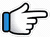# Abhi Mujh Mein Kahin – Keyboard Notes## Abhi Mujh Mein Kahin Keyboard NotesFor Abhi Mujh Mein Kahin Notes as Western or Indian Notations, Click –

Western –
Indian –

## Keyboard Notes for Abhi Mujh Mein Kahin – Indian Notation (srg mpdn)

Md~~    ndMg~          Md~~
Abhi~    mujh_mein   kahin~~
Or
Md~~    ndpM~          pd~~

nd  pM     p~ n     d
Baa~ki     thodi    si

Mpn   p~ M M~~
hai     zindagi

Md~~    ndMg~     Md~~
Or
Md~~   ndpM~     pd~~

ndpM     p~ n     d
Jaa_na   zinda   hoon

Mp      n      p~M
main   toh   abhi~
Or
Mpn   p~    M M

Md     n~ R*    r*R*n
Kuch   aisi       lagan
Or
Md     n~ R*    r*~ n~

Md   n~ R*    r*        R*n
iss     lamhe  mein   hai

Md   n~ R*     r*n         R*   r*g*~~
Ye     lamha   kahaan   tha   mera~~

r*g*    M*~   g*~ r*R*n
Ab       hai      saamne

r*g*     M*~     g*~     r*R*n
Issey    chhoo   loon   zaraa

R*r*     n~ r*~
Mar      jaaoon

p*   M*g*    r*     R*r*~
ya   jee        loon   zaraa~~

r*g*M*~        g*~  r* R*n
Khushiyaan   choom _  loon

d*p*    M*~   g*~    r* R*n
Yaa      ro       loon   zaraa
Or
r*g*    M*~   g*~    r* R*n

R*r*     n~ r*~
Mar      jaaoon

p*   M*g*   r*     R*r*~
ya   jee       loon   zaraa~~

R*ndp
Ho..   o..

Md~~     ndMg~          Md~~
Abhi~    mujh_mein   kahin~~

nd  pM      p~ n     d
Baa~ki      thodi    si

Mpn   p~ M M~~
hai     zindagi

R*….
Ho..

r*~ R*     d
dhoop     mein

n~ s*   np      Mp      d
jalte    huey   tann   ko,

d~ n~    Mn   N   Mg   Md~
chhaya   ped   ki    mill   gayee

r*~ R*     d~ n         s*
Roothe   bachche   ki

np         Mpd
hansi     jaise~~

dnM~ n        N    M      g         Md~
Phuslaane  se   phir  khill   gayee~

rg          d~ Dd
Kuchh  aisa_hi

Dd     DdD~d        Dd    Dd
abb   mehsoos    dil     ko

p     MgM    r
ho   rahaa   hai

rgd~         DdDd               DdD~d
Barso’n  ke_puraane   zakhm_pe

nR*r*~      R*n    pd    MM
marham   laga   saa    hai

Md      n~ R*  r*R*n
Kuch   aisa     rahem,

Md   n~ R*~    r*   n
iss    lamhe    mein_hai

Md   n~ R*    r*n          R*      r*g*~~
Ye    lamha   kahaan  tha   me~ra~~

r*g*    M*~   g*~ r*R*n
Ab        hai      saamne

r*g*      M*~     g*~     r*R*n
Issey    chhoo   loon   zaraa

R*r*     n~ r*~
Mar      jaaoon

p*   M*g*    r*     R*r*~
ya   jee         loon   zaraa~~

r*g*M*~        g*~  r* R*n
Khushiyaan   choom _  loon

d*p*   M*~   g*~    r* R*n
Yaa      ro       loon   zaraa
Or
r*g*   M*~   g*~    r* R*n

R*r*     n~ r*~
Mar      jaaoon

p*   M*g*    r*     R*r*~
ya   jee         loon   zaraa~~

Will be Updated:
Dor se tooti patang jaisi,
thi ye zindagani meri

Aaj ho kal ho mera naa ho
Har din thi kahani meri

Ik bandhan naya
peechhe se abb mujhko bulaaye
Aane waley kal ki kyun
fikr mujhko sata jaaye

Ik aisi chubhan iss lamhe me hai
Ye lamha kahaan tha mera aa

r*g*    M*~   g*~ r*R*n
Ab        hai      saamne

r*g*      M*~     g*~     r*R*n
Issey    chhoo   loon   zaraa

R*r*     n~ r*~
Mar      jaaoon

p*   M*g*    r*     R*r*~
ya   jee         loon   zaraa~~

r*g*M*~        g*~  r* R*n
Khushiyaan   choom _  loon

d*p*   M*~   g*~    r* R*n
Yaa      ro       loon   zaraa
Or
r*g*   M*~   g*~    r* R*n

R*r*     n~ r*~
Mar      jaaoon

p*   M*g*    r*     R*r*~
ya   jee         loon   zaraa~~